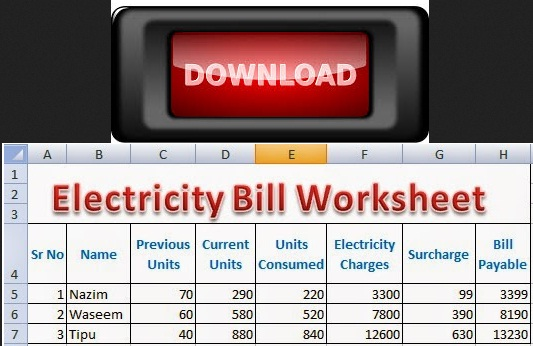# Perfect Computer Notes

Super Easy Short Computer Notes To Improve Computer Literacy and Your IT Skills

# Electricity Bill Calculator Formulas in Excel

Today, we will discuss How to create an Electricity Bill Calculator Worksheet by using Electricity Bill Calculator Formulas in Excel. The following formulas will be used in Electricity Bill Calculator Worksheet:

## Q: Prepare a worksheet according to the following instructions:

1. Create a worksheet in MS Excel according to the requirements
2. Enter sample data of Electricity units consumed by customers in a city
3. Apply formula to calculate Units Consumed
4. Apply formula to calculate Electricity Charges
5. Apply formula to calculate Surcharge
6. Apply formula to calculate Bill Payable
7. Note: Every formula will start from  =
 Units Consumed Formula=D5-C5 Electricity Charges Formula=IF(E5>200, E5*15,IF(E5>100, 1000+(E5-100)*12, E5*10)) SURCHARGE FORMULA = IF(F5>5000, F5*5%,IF(F5>1000, F5*3%, 0)) BILL PAYABLE FORMULA = SUM(F5:G5)Download Excel Electricity Bill Calculation Worksheet Free

## Image for Electricity Bill Calculator Formulas in Excel Software

Solution:
1. Create a new workbook in MS Excel and save as with the name Electricity Bill Calculator Formulas in Excel
2. Enter sample data for at least three customers
3. Enter formula for Units Consumed= D5 - C5 in cell with cell reference E5.
4. Enter formula for Electricity Charges =IF(E5>200, E5*15,IF(E5>100, 1000+(E5-100)*12, E5*10)) in cell with cell reference F5.
5. Enter formula for Surcharge = IF(F5>5000, F5*5%,IF(F5>1000, F5*3%, 0)) in cell with cell reference G5.
6. Enter formula for Bill Payable =SUM(F5:G5) in cell with cell reference H5.
So, the worksheet with Electricity Bill Calculator Formulas in Excel Worksheet has been prepared successfully.